# Voltage Sensor: Working Principle, Types & Circuit Diagram

Contents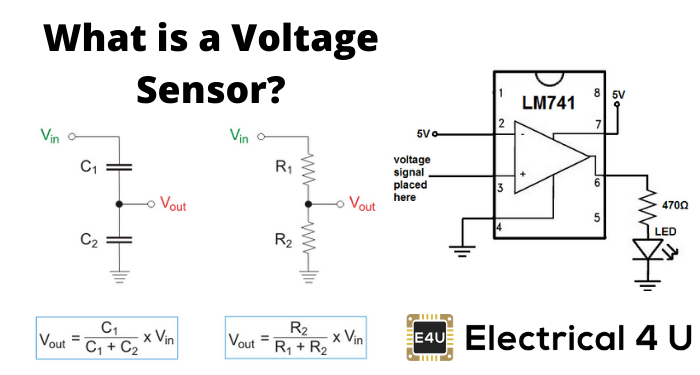## What is a Voltage Sensor?

A voltage sensor is a sensor used to calculate and monitor the amount of voltage in an object. Voltage sensors can determine the AC voltage or DC voltage level. The input of this sensor is the voltage, whereas the output is the switches, analog voltage signal, a current signal, or an audible signal.

Sensors are devices that can sense or identify and react to certain types of electrical or optical signals. The implementation of a voltage sensor and current sensor techniques have become an excellent choice for the conventional current and voltage measurement methods.

## Types of Voltage Sensors

In this article, we can discuss a voltage sensor in detail. A voltage sensor can determine, monitor, and measure the supply of voltage. It can measure the AC level and/or DC voltage level. The input to the voltage sensor is the voltage itself, and the output can be analog voltage signals, switches, audible signals, analog current levels, frequency, or even frequency-modulated outputs.

That is, some voltage sensors can provide sine or pulse trains as output, and others can produce amplitude modulation, pulse width modulation, or frequency modulation outputs.

In voltage sensors, the measurement is based on a voltage divider. Two main types of voltage sensors are available: capacitive type voltage sensor and resistive type voltage sensor.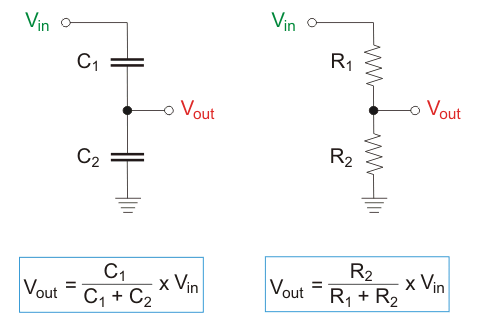### Capacitive Voltage Sensor

We know that a capacitor comprises of two conductors (or two plates); between these plates, a non-conductor is kept.

That non-conducting material is termed dielectric. When an AC voltage is provided across these plates, current will start to pass due to either the electrons’ attraction or repulsion via the opposite plate’s voltage.

The field among the plates will create a complete AC circuit without any hardware connection. This is how a capacitor works.

Next, we can discuss the voltage division in two capacitors which are in series. Usually, in series circuits, high voltage will develop across the component with high impedance. In the case of capacitors, capacitance and impedance (capacitive reactance) are always inversely proportional.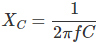The relation between voltage and capacitance is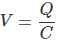Q → Charge (Coulomb)
XC → Capacitive reactance (Ω)
f → Frequency (Hertz)

From the above two relations, we can clearly state that the highest voltage will accumulate across the smallest capacitor. The capacitor voltage sensors work based on this simple principle. Consider we are holding the sensor and then placing its tip near a live conductor.

Here, we are inserting the sensing element of high impedance into a series capacitive coupling circuit.

Presently, the sensor’s tip is the smallest capacitor coupled to the live voltage. Thus, the whole voltage will develop across the sensing circuit, which can detect voltage, and the light or buzzer indicator is turned on—this is behind the non-contact voltage sensors you use at home.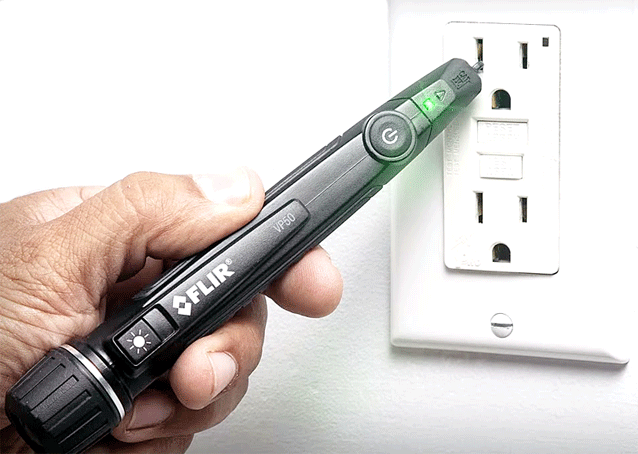### Resistive Voltage Sensor

Two ways exist to convert the resistance of the sensing element to the voltage. The first one is the simplest method, which is to provide a voltage to the resistor divider circuit comprised of a sensor and a reference resistor, which is represented below.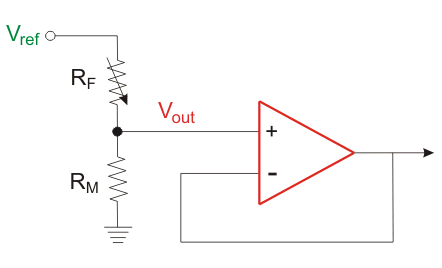The voltage developed across the reference resistor or sensor is buffered and then given to the amplifier. The sensor’s output voltage can be expressed as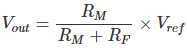This circuit’s drawback is that the amplifier present will amplify the whole voltage developed across the sensor. However, it is better to amplify only the voltage change due to the change in the sensor’s resistance, which is achieved by the second method implementing the resistance bridge, as shown below.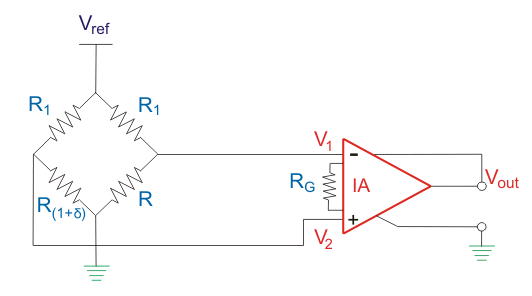Here, the output voltage is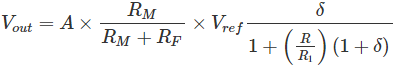When R1 = R, then the output voltage becomes approximately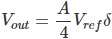A → Gain of instrumentation amplifier
δ → Change in the resistance of the sensor, which is analogous to some physical action

In this equation, the gain must be set high because only the voltage change due to the change in the sensor’s resistance is being amplified.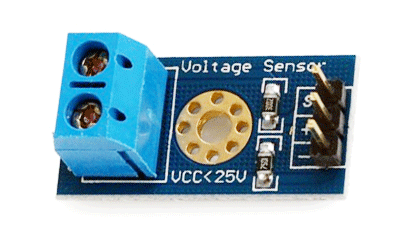## Advantages of Voltage Sensors Over Conventional Measuring Techniques

The advantage of voltage sensors include:

• Small in weight and size
• Personnel safety is high
• The degree of accuracy is very high
• Non-saturable
• Wide dynamic range
• Eco-friendly

Can combine the voltage and current measurement into a single physical device with small and compact dimensions

## Applications of Voltage Sensors

The application of voltage sensors include the following:

• Power failure detection
• Safety switching
• Temperature control
• Power demand control
• Fault detection

Voltage sensors are included in many of the best Arduino starter kits, as they are very useful in many electronics projects.

Want To Learn Faster? 🎓
Get electrical articles delivered to your inbox every week.
No credit card required—it’s 100% free.